# 网络流

## 最大流

### 定义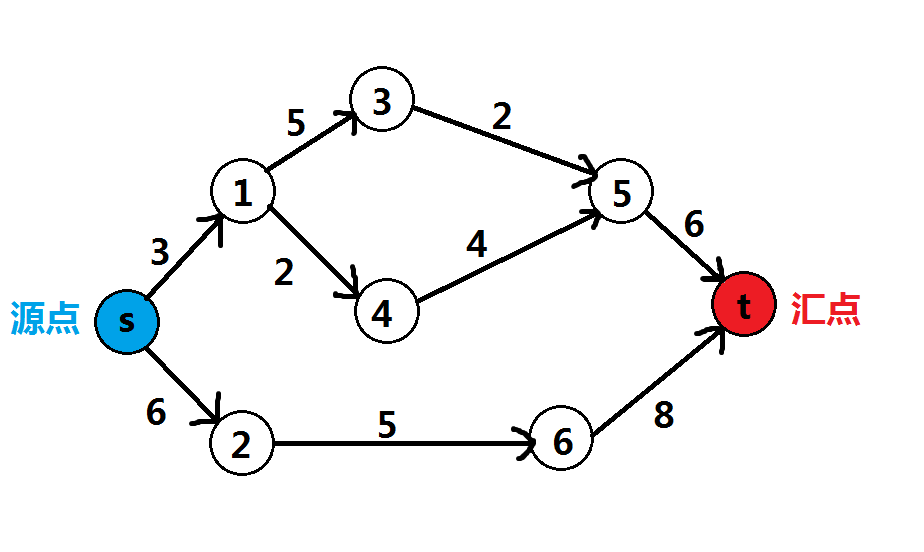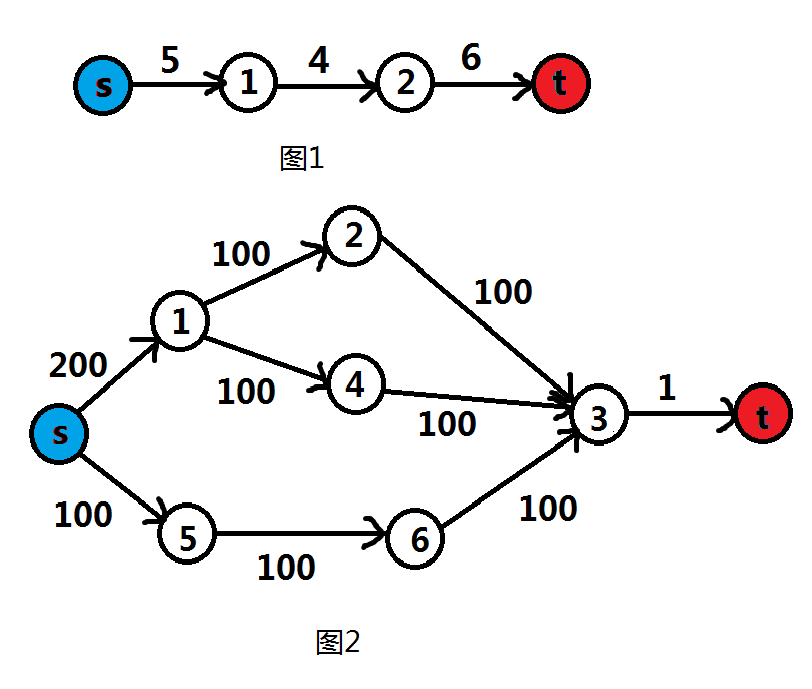### 求最大流的算法

#### EK（Edmond—Karp）算法

• 增广路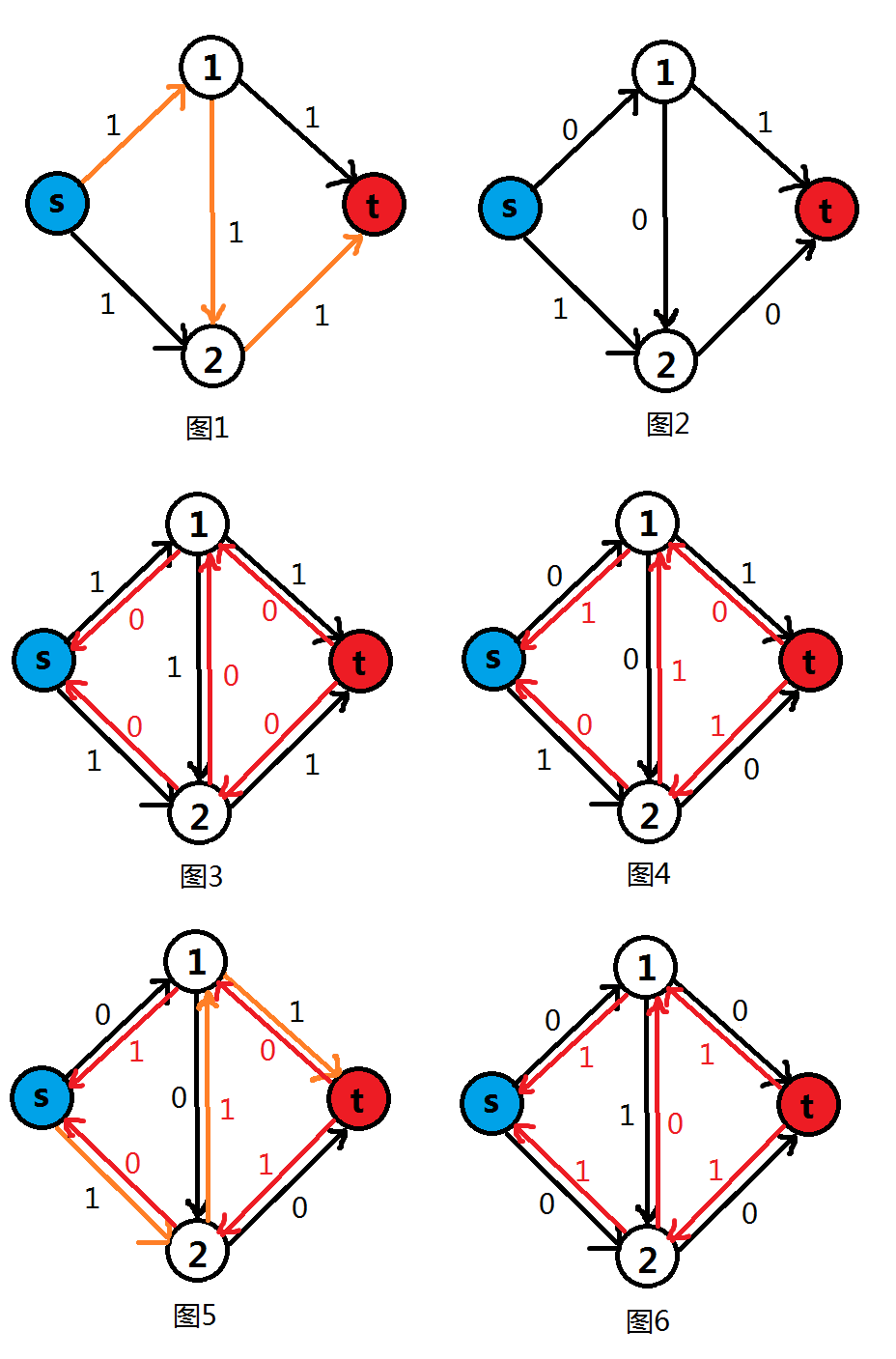$$\color{red}{给用过的边做一个标记，找到更好的情况时可以把以前的情况给“撤销”了}$$

• 代码

P3376 【模板】网络最大流 为例

1. 建图
建图的方法很多，我比较喜欢采用邻接表（链式前向星）存储：
struct node{//结构体
int to,dis,nex;
//to:边指向的节点 dis:权值/容量 nex:同起点的下一条边的下标
}a[maxm*2];
a[++num].to=to;
a[num].dis=dis;
}

1. $$BFS$$找增广路
从源点$$s$$开始，$$BFS$$搜每个节点，当且仅当边权（即容量）$$>0$$时进入，到达汇点$$t$$时即为一条增广路。
bool bfs(){
queue<int> q;//队列
memset(vis,0,sizeof(vis));
vis[s]=1;//是否搜过
q.push(s);
while(!q.empty()){
int top=q.front();//队首
q.pop();
if(!vis[a[i].to]&&a[i].dis){//若没去过且容量大于0
vis[a[i].to]=1;//标记去过
p[a[i].to].pre=top;//记录路径,pre为上一个点
p[a[i].to].edge=i;//记录路径,edge为边
if(a[i].to==t){//到汇点返回1,表示有增广路
return 1;
}
q.push(a[i].to);
}
}
}
return 0;//若全部搜完还没有出现增广路,返回0
}

1. $$EK$$算法核心
不断$$BFS$$搜增广路，更新权值。搜不到增广路时答案即为最大流。
• 特别注意，代码中将下标异或$$1$$后得到的即为其反向边。因为建边时边和其反向边总是成对出现且反向边为奇数。
void ek(){
long long ans=0;
while(bfs()){//不断找增广路
int minn=99999999;
for(int i=t;i!=s;i=p[i].pre){
minn=min(minn,a[p[i].edge].dis);//找最小容量
}
for(int i=t;i!=s;i=p[i].pre){//更改
a[p[i].edge].dis-=minn;
a[p[i].edge^1].dis+=minn;
}
ans+=minn;
}
printf("%lld",ans);//输出最大流
}

1. 完整代码
#include<iostream>
#include<cstdio>
#include<cstring>
#include<queue>
#define maxn 210
#define maxm 5005
using namespace std;
int n,m,s,t,u,v,w;
struct node{
int to,dis,nex;
}a[maxm*2];
a[++num].to=to;
a[num].dis=dis;
}
bool vis[maxn];
struct path{
int pre,edge;
}p[maxm*2];
bool bfs(){
queue<int> q;
memset(vis,0,sizeof(vis));
vis[s]=1;
q.push(s);
while(!q.empty()){
int top=q.front();
q.pop();
if(!vis[a[i].to]&&a[i].dis){
vis[a[i].to]=1;
p[a[i].to].pre=top;
p[a[i].to].edge=i;
if(a[i].to==t){
return 1;
}
q.push(a[i].to);
}
}
}
return 0;
}
void ek(){
long long ans=0;
while(bfs()){
int minn=99999999;
for(int i=t;i!=s;i=p[i].pre){
minn=min(minn,a[p[i].edge].dis);
}
for(int i=t;i!=s;i=p[i].pre){
a[p[i].edge].dis-=minn;
a[p[i].edge^1].dis+=minn;
}
ans+=minn;
}
printf("%lld",ans);
}
int main(){
scanf("%d%d%d%d",&n,&m,&s,&t);
for(int i=1;i<=m;i++){
scanf("%d%d%d",&u,&v,&w);
}
ek();
return 0;
}


#### Dinic算法

Dinic算法（又称Dinitz算法）是一个在网络流中计算最大流的强多项式复杂度的算法，设想由以色列（前苏联）的计算机科学家Yefim (Chaim) A. Dinitz在1970年提出。

$$Dinic$$算法的过程如下：

1. $$BFS$$将图分层；
2. $$DFS$$搜出所有增广路；
3. 重复前两步，直到找不到增广路为止。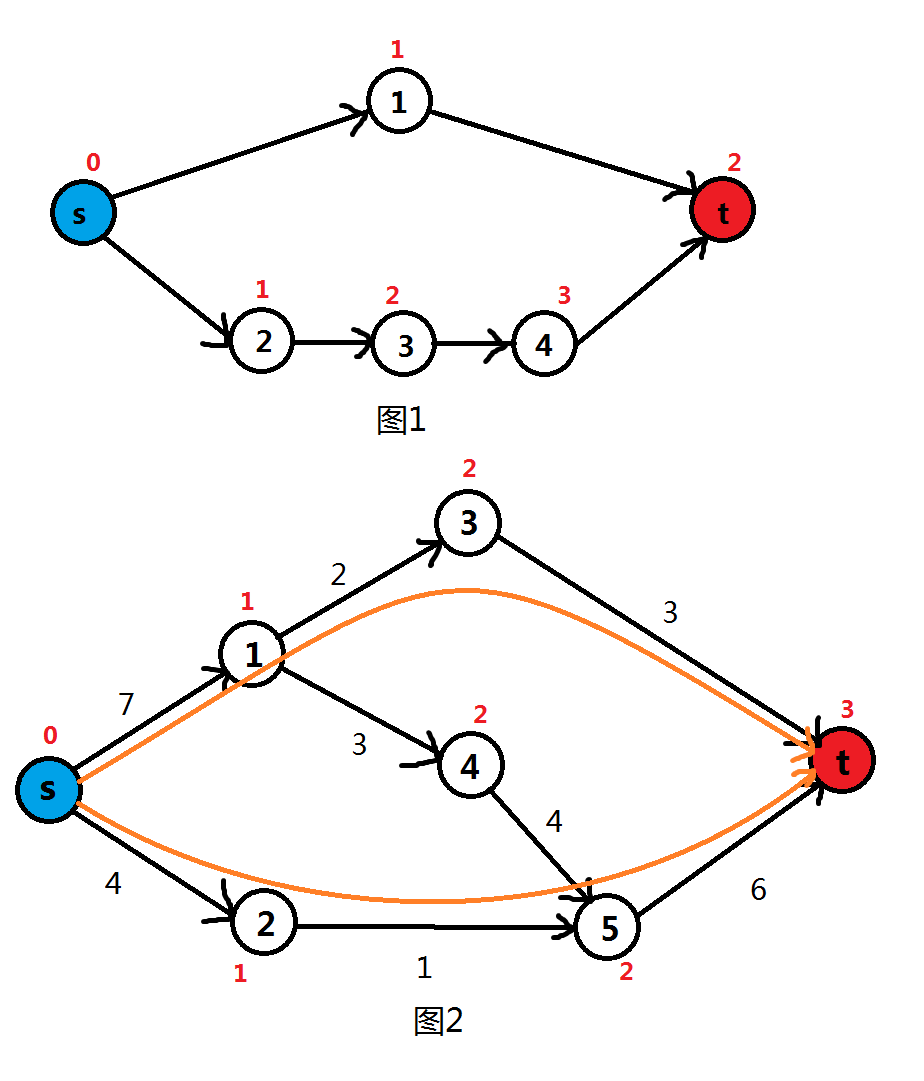• 代码（例题还是一样的）
1. $$BFS$$分层
$$BFS$$时，先将源点$$s$$的层数设为$$0$$$$1$$其实也没什么关系），之后广搜，找到能更新的边就更新。
bool bfs(){
memset(dep,0x3f,sizeof(dep));//层数
memset(vis,0,sizeof(vis));//是否入过队
queue<int> q;
dep[s]=0;
q.push(s);
vis[s]=1;
while(!q.empty()){
int top=q.front();
q.pop();
if(dep[a[i].to]>dep[top]+1&&a[i].dis){//可更新的就更新
dep[a[i].to]=dep[top]+1;
if(!vis[a[i].to]){//没入过队的入队
vis[a[i].to]=1;
q.push(a[i].to);
}
}
}
}
if(dep[t]==dep){//若汇点t的层数和0号节点一样,即为初始值,意味着没有增广路了,返回false
return 0;
}
return 1;
}

1. $$DFS$$搜索
$$minn$$表示这条路的最大容量（其实就是上面介绍$$EK$$时的$$min$$），$$use$$表示用过的容量。$$use$$的主要作用是，记录当前搜过的增广路要减去的流量，当$$use$$的值等于$$minn$$时，意味着当前的边已经达到了最大容量，此时停止搜索。
ll dfs(int x,int minn){//x为当前节点编号
if(x==t){//到达汇点
ans+=minn;//累加答案
return minn;
}
int use=0;
if(a[i].dis&&dep[a[i].to]==dep[x]+1){//满足最短增广路且还有剩余容量
int nex=dfs(a[i].to,min(minn-use,a[i].dis));//向下搜
if(nex>0){//若结果大于0,说明有增广路,因为若下面没有增广路了则会返回use的初值0
use+=nex;//增加当前流量
a[i].dis-=nex;
a[i^1].dis+=nex;
if(use==minn){//达到最大容量
break;
}
}
}
}
return use;
}

1. $$Dinic$$算法核心
非常简洁，因为主要部分都在两个搜索里了。
void dinic(){
while(bfs()){//若有增广路
dfs(s,99999999);//搜索(minn的初值大一点就好)
}
printf("%lld",ans);//搜完输出答案
}

1. 完整代码
#include<iostream>
#include<cstdio>
#include<cstring>
#include<queue>
#define maxn 210
#define maxm 5005
#define ll long long
using namespace std;
int n,m,s,t,u,v,w;
ll ans=0;
struct node{
int to,dis,nex;
}a[maxm*2];
a[++num].to=to;
a[num].dis=dis;
}
bool vis[maxn];
int dep[maxn];
bool bfs(){
memset(dep,0x3f,sizeof(dep));
memset(vis,0,sizeof(vis));
queue<int> q;
dep[s]=0;
q.push(s);
vis[s]=1;
while(!q.empty()){
int top=q.front();
q.pop();
if(dep[a[i].to]>dep[top]+1&&a[i].dis){
dep[a[i].to]=dep[top]+1;
if(!vis[a[i].to]){
vis[a[i].to]=1;
q.push(a[i].to);
}
}
}
}
if(dep[t]==dep){
return 0;
}
return 1;
}
ll dfs(int x,int minn){
if(x==t){
ans+=minn;
return minn;
}
int use=0;
if(a[i].dis&&dep[a[i].to]==dep[x]+1){
int nex=dfs(a[i].to,min(minn-use,a[i].dis));
if(nex>0){
use+=nex;
a[i].dis-=nex;
a[i^1].dis+=nex;
if(use==minn){
break;
}
}
}
}
return use;
}
void dinic(){
while(bfs()){
dfs(s,99999999);
}
printf("%lld",ans);
}
int main(){
scanf("%d%d%d%d",&n,&m,&s,&t);
for(int i=1;i<=m;i++){
scanf("%d%d%d",&u,&v,&w);
}
dinic();
return 0;
}


#### Dinic算法+当前弧优化

• 代码（最终版本）

#include<iostream>
#include<cstdio>
#include<cstring>
#include<queue>
#define maxn 205
#define maxm 5005
#define ll long long
#define inf 0x3fffffff
using namespace std;
int n,m,s,t,u,v,w;
struct node{
int to,dis,nex;
}a[maxm*2];
a[++tt].to=to;
a[tt].dis=dis;
}
bool vis[maxn];
int dep[maxn],cur[maxn];
bool bfs(){
for(int i=0;i<=n;i++){
vis[i]=0;
dep[i]=inf;
}
queue<int> q;
vis[s]=1;
q.push(s);
dep[s]=0;
while(!q.empty()){
int top=q.front();
q.pop();
if(dep[top]+1<dep[a[i].to]&&a[i].dis){
dep[a[i].to]=dep[top]+1;
if(!vis[a[i].to]){
vis[a[i].to]=1;
q.push(a[i].to);
}
}
}
}
if(dep[t]==dep){
return 0;
}
return 1;
}
ll ans=0;
int dfs(int x,int minn){
if(x==t){
ans+=minn;
return minn;
}
int use=0;
for(int i=cur[x];i;i=a[i].nex){
cur[x]=i;
if(dep[a[i].to]==dep[x]+1&&a[i].dis){
int search=dfs(a[i].to,min(minn-use,a[i].dis));
if(search>0){
use+=search;
a[i].dis-=search;
a[i^1].dis+=search;
if(use==minn){
break;
}
}
}
}
return use;
}
void dinic(){
while(bfs()){
dfs(s,inf);
}
printf("%lld",ans);
}
int main(){
scanf("%d%d%d%d",&n,&m,&s,&t);
for(int i=1;i<=m;i++){
scanf("%d%d%d",&u,&v,&w);
}
dinic();
return 0;
}


## 最小割

### 定义

，汇点$$t\in T$$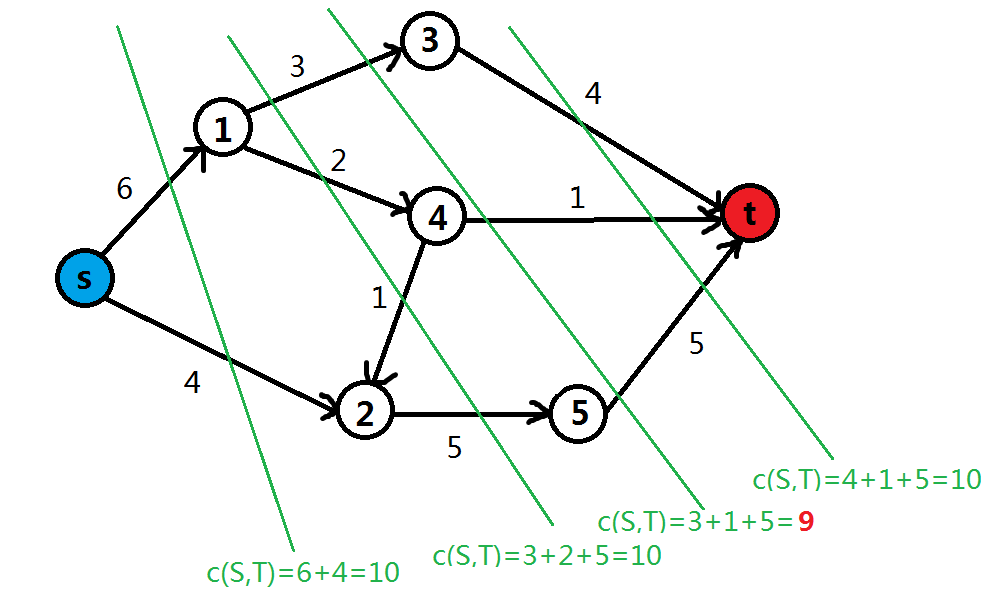### 最大流最小割定理

#### 定理内容

1. 如果$$f$$是网络中的一个流，$$c(S,T)$$是任意一个割，那么$$f$$等于正向割边的流量与负向割边的流量之差。
2. $$f(s,t)_{max}=c(s,t)_{min}$$，即最大流等于最小割

#### 证明

1. 设两点集分别为$$S$$$$T$$，定义$$c(A,B)$$表示从$$A$$指向$$B$$的边的容量和（其实就是割的容量）。则只需证明$$f=c(S,T)-c(T,S)$$即可。
显然若$$B_1\cap B_2=\varnothing$$，则$$c(A,(B_1\cup B_2))=c(A,B_1)+c(A,B_2)$$$$c((B_1\cup B_2),A)=c(B_1,A)+c(B_2,A)$$$$\quad ①$$
那么若有一个节点$$X\in S$$
$$X$$为源点，则$$c(X,S\cup T)-c(S\cup T,X)=f$$
$$X$$不是源点，则$$c(X,S\cup T)-c(S\cup T,X)=0$$
因为点集$$S$$中所有点都满足上述关系式，相加得到$$c(S,S\cup T)-c(S\cup T,S)=f$$。用①式得到：
$f=c(S,S\cup T)-c(S\cup T,S)=(c(S,S)+c(S,T))-(c(S,S)+c(T,S))=c(S,T)-c(T,S)$

1. $$1$$得到，对于每一个可行的流$$f(s,t)$$和一个割$$(S,T)$$，我们可以得到$$f(s,t)=c(S,T)-c(T,S)\le c(S,T)$$
特别地，当$$f$$为最大流时，原图中一定没有增广路，即$$S$$的出边一定满流，入边一定零流，即$$f(s,t)=c(S,T)$$
由以上两个式子得到此时$$f$$一定最大，$$c$$一定最小。即此时$$f$$为最大流，$$c$$为最小割，最大流等于最小割。

## 费用流—最小费用最大流

### 定义

$$Dinic$$一样，我们要分层、搜增广路并更新。那么，我们应该更改哪一步呢？

### 代码

#include<iostream>
#include<cstdio>
#include<cstring>
#include<queue>
#define maxn 5005
#define maxm 50005
#define ll long long
#define inf 0x3fffffff
using namespace std;
int n,m,s,t,u,v,w,cost;
struct node{
int to,dis,cost,nex;
}a[maxm*2];
void add(int from,int to,int dis,int cost){
a[++tt].to=to;
a[tt].dis=dis;
a[tt].cost=cost;
}
bool vis[maxn];
int costs[maxn];
bool spfa(){
memset(vis,0,sizeof(vis));
memset(costs,0x3f,sizeof(costs));
queue<int> q;
vis[s]=1;
q.push(s);
costs[s]=0;
while(!q.empty()){
int top=q.front();
q.pop();
if(costs[top]+a[i].cost<costs[a[i].to]&&a[i].dis){
costs[a[i].to]=costs[top]+a[i].cost;
if(!vis[a[i].to]){
vis[a[i].to]=1;
q.push(a[i].to);
}
}
}
}
if(costs[t]==costs){
return 0;
}
return 1;
}
ll ans=0,anscost=0;
int dfs(int x,int minn){
if(x==t){
vis[t]=1;
ans+=minn;
return minn;
}
int use=0;
vis[x]=1;
if((!vis[a[i].to]||a[i].to==t)&&costs[a[i].to]==costs[x]+a[i].cost&&a[i].dis){
int search=dfs(a[i].to,min(minn-use,a[i].dis));
if(search>0){
use+=search;
anscost+=(a[i].cost*search);
a[i].dis-=search;
a[i^1].dis+=search;
if(use==minn){
break;
}
}
}
}
return use;
}
void dinic(){
while(spfa()){
vis[t]=1;
while(vis[t]){
memset(vis,0,sizeof(vis));
dfs(s,inf);
}
}
printf("%lld %lld",ans,anscost);
}
int main(){
scanf("%d%d%d%d",&n,&m,&s,&t);
for(int i=1;i<=m;i++){
scanf("%d%d%d%d",&u,&v,&w,&cost);